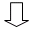### Manipulating the UI in loops

Excessive and unnecessary loops have a negative impact on performance. Some PowerBuilder code will trigger your Appeon Web application to redraw visual objects, such as DataWindows, controls, etc. If such functions are put into a loop, it will redraw the visual objects numerous times and therefore negatively affecting performance.

Appeon recommends the following:

• Do not put functions that operate on DataWindow rows into a loop.

• Avoid placing functions that result in the repaint of visual control(s) into a loop; otherwise, the visual control(s) will be repainted many times while the loop is executed.

• Use the Find function for DataWindow search instead of using the loop statement.

The following is an example:

```Long ll_row
String ls_expression
String ls_Name
ls_Name = "Mike"
For ll_row = 1 To dw_1.RowCount()
ls_expression =
dw_1.GetItemString(ll_row,"name")
If ls_expression = ls_Name Then

Exit
End If
Next``````Long ll_row
Long ll_rowcount
String ls_expression
String ls_Name
ls_Name = "Mike"
ll_rowcount = dw_1.RowCount()
For ll_row = 1 To ll_rowcount
ls_expression = +
dw_1.GetItemString(ll_row,"name")
If ls_expression = ls_Name Then
...
Exit
End If
Next``````Long ll_row
Long ll_rowcount
String ls_expression
String ls_Name
ls_Name = "Mike"
ll_rowcount = dw_1.RowCount()
ls_expression = "name = '" + ls_Name + "'"
ll_row = dw_1.Find(ls_expression,1,ll_rowcount)
...```